### Home > MC2 > Chapter 9 > Lesson 9.3.2 > Problem9-94

9-94.

Find the missing dimension on each rectangle or square. Homework Help ✎

 a.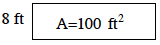b.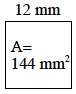c.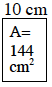d.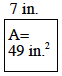e.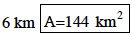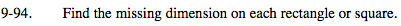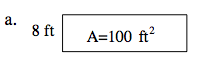If area is length times width, then:
8(x) = 100

12.5 ft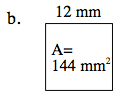What are the characteristics of a square?

The side lengths of a square are all equal.

12 (12) = 144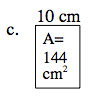See part (a).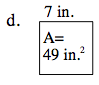See part (b).

7 in.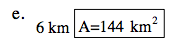See part (a).

24 km